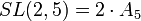# Supergroups of alternating group:A5

View supergroups of particular groups | View other specific information about alternating group:A5

This article discusses some of the groups that admit the alternating group of degree five as a subgroup, quotient group, or subquotient.

Note that unlike the discussion of the subgroup structure of alternating group:A5, this discussion is necessarily not comprehensive, because there are infinitely many groups containing the alternating group of degree five.

## Subgroups and quotients: essential minimalist examples

### Subgroups: making all automorphisms inner

Further information: symmetric group:S5, A5 in S5

The outer automorphism group of alternating group:A5 is cyclic group:Z2, and the whole automorphism group is symmetric group:S5. Since alternating group:A5 is a centerless group, it embeds as a subgroup of index two inside its automorphism group, which is symmetric group on five elements.$A_5$ is a simple non-abelian group and$A_5$ and$S_5$ are the only two almost simple groups corresponding to$A_5$.$A_5$ is also of index two in the full icosahedral group, which turns out not to be$S_5$, but instead the direct product of$A_5$ and the cyclic group of order two.

### Quotients: Schur covering groups

Further information: special linear group:SL(2,5), center of special linear group:SL(2,5)

The Schur multiplier of$A_5$ is cyclic group:Z2.

The corresponding universal central extension (the unique Schur covering group, unique because$A_5$ is a perfect group) is special linear group:SL(2,5), also denoted as$2 \cdot A_5$ to denote that it is a double cover (see double cover of alternating group). The center of special linear group:SL(2,5) is cyclic group:Z2 and the quotient group is$A_5$.$A_5$ is a simple non-abelian group and$A_5$ and$SL(2,5) = 2 \cdot A_5$ are the only two corresponding quasisimple groups.